Question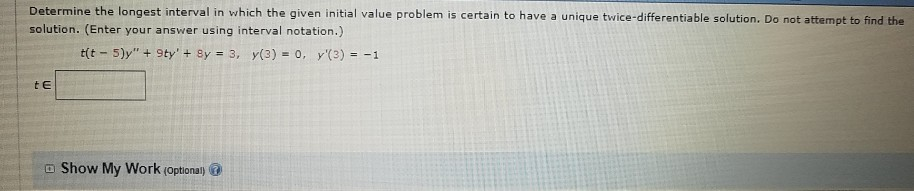Determine the longest interval in which the given initial value problem is certain to have a unique twice-differentiable solution. Do not a solution. (Enter your answer using interval notation.) t E 匝 Show My Work@ptionali@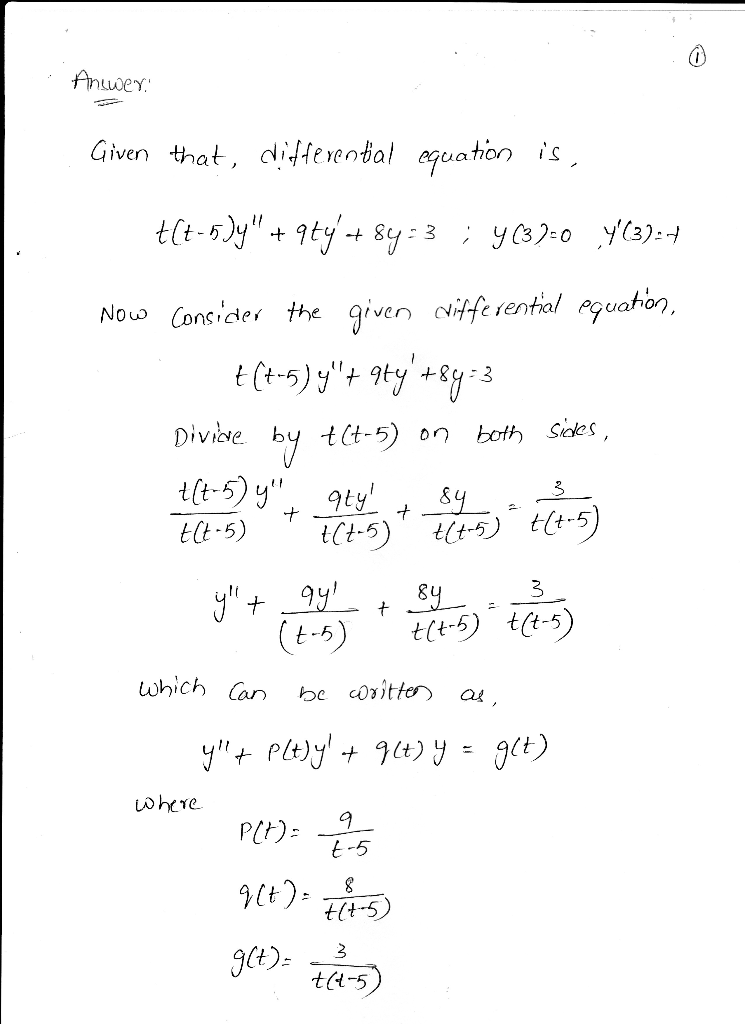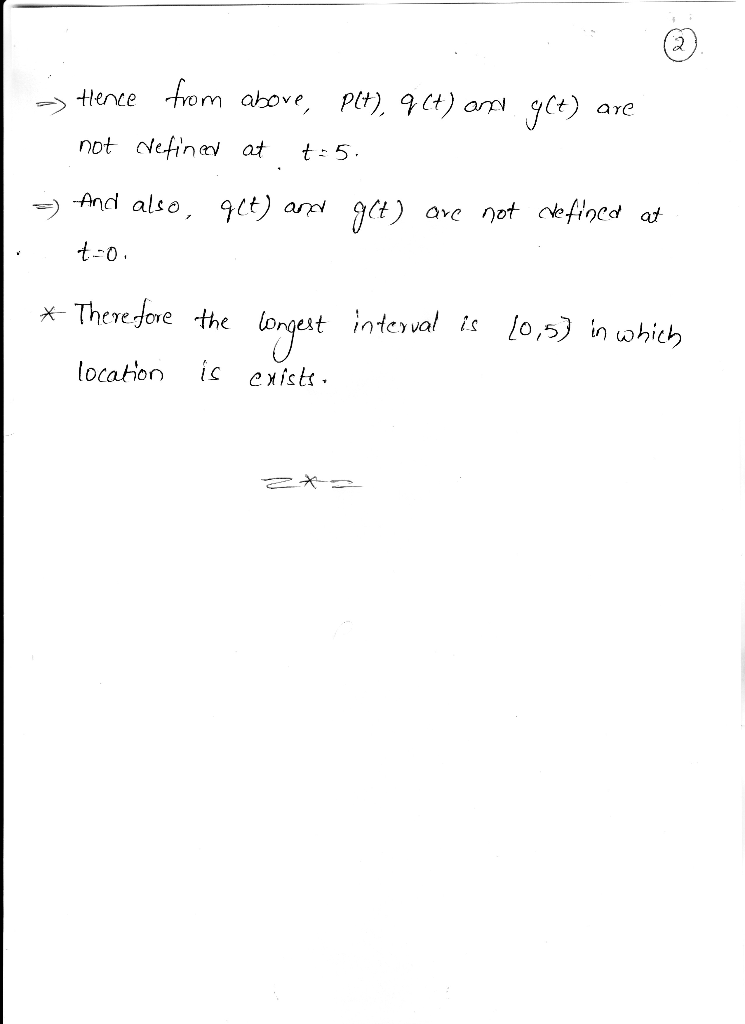#### Earn Coins

Coins can be redeemed for fabulous gifts.

Similar Homework Help Questions
• ### State the longest interval, if any, in which the given IVP is certain to have a...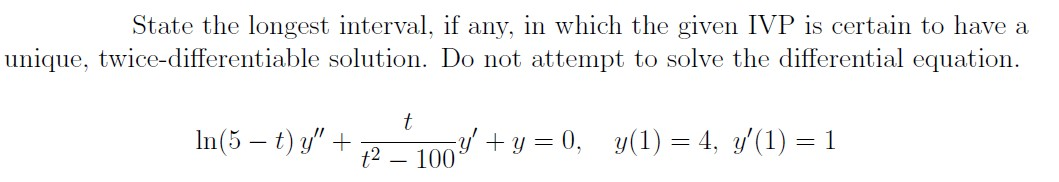State the longest interval, if any, in which the given IVP is certain to have a unique, twice-differentiable solution. Do not attempt to solve the differential equation. t In(5 – t) y" + - 100V t2 fy' +y = 0, y(1) = 4, y'(1) = 1 - 100

• ### Problem 4 ( 14 points) (a) Determine the longest interval in which the given initial value...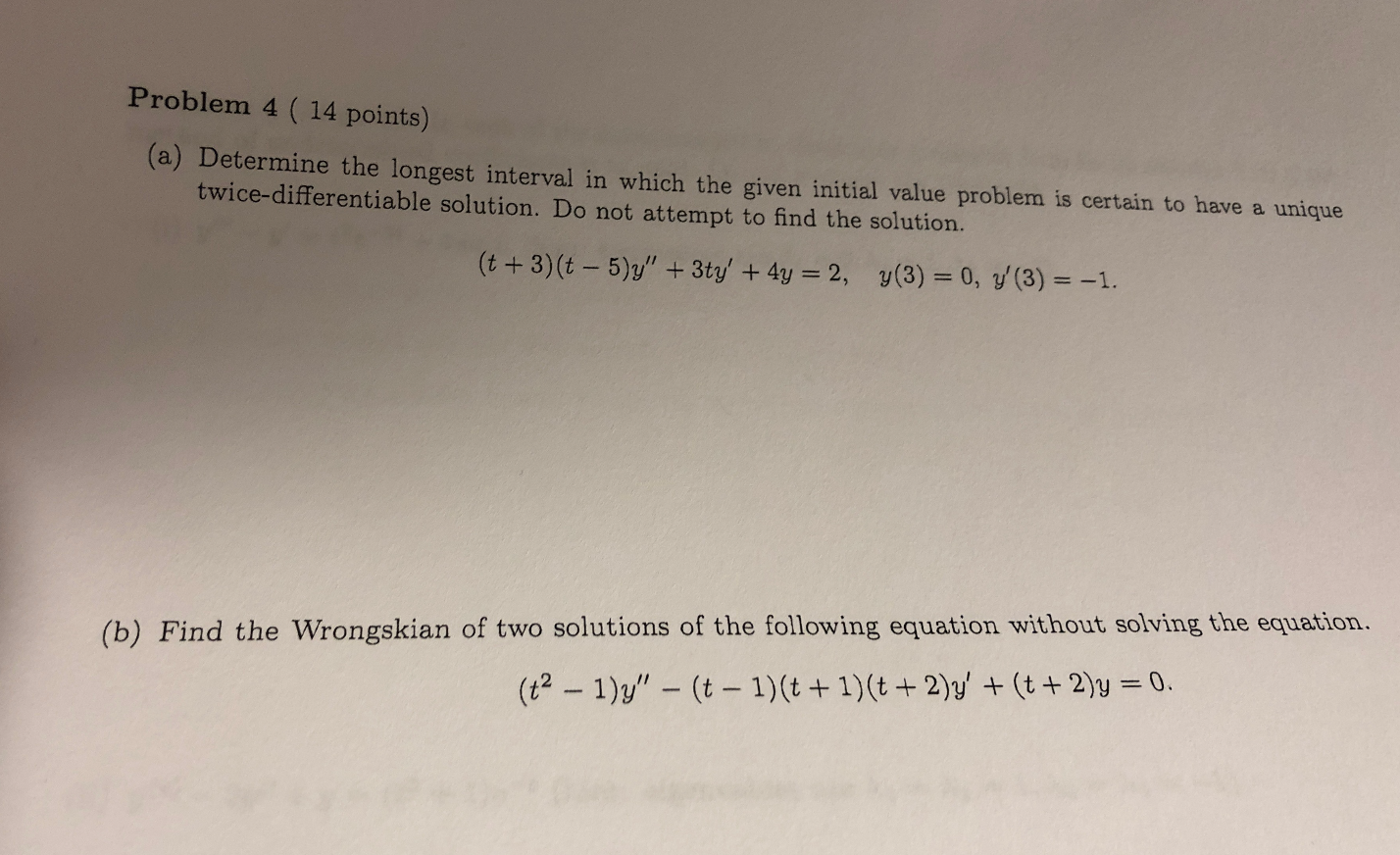Problem 4 ( 14 points) (a) Determine the longest interval in which the given initial value problem is certain to have a unique twice-differentiable solution. Do not attempt to find the solution. (t +3)(t - 5)/" + 3ty' + 4y = 2, y(3) = 0, y(3) = -1. (b) Find the Wrongskian of two solutions of the following equation without solving the equation. (t2 – 1)y" – (t – 1)(t + 1)(t + 2)y' + (t + 2)y = 0.

• ### Problem 4 ( 14 points) (a) Determine the longest interval in which the given initial value...Problem 4 ( 14 points) (a) Determine the longest interval in which the given initial value problem is certain to have a unique twice-differentiable solution. Do not attempt to find the solution. (t +3)(t - 5)/" + 3ty' + 4y = 2, y(3) = 0, y(3) = -1. (b) Find the Wrongskian of two solutions of the following equation without solving the equation. (t2 – 1)y" – (t – 1)(t + 1)(t + 2)y' + (t + 2)y = 0.

• ### 4. Determine the longest interval in which the initial value problem below is certain to have...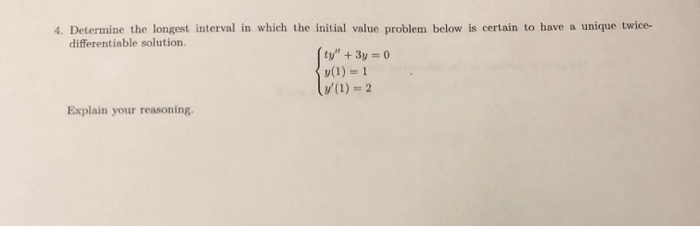4. Determine the longest interval in which the initial value problem below is certain to have a unique twice- differentiable solution. ty"+3y 0 y(1) 1 (1) = 2 Explain your reasoning.

• ### Chapter 3, Section 3.2, Additional Go Tutorial Problem 02 11 Determine the longest interval in which...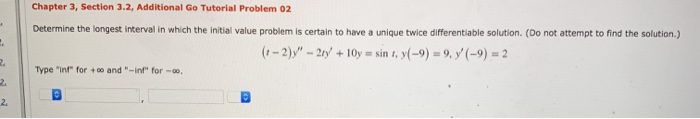Chapter 3, Section 3.2, Additional Go Tutorial Problem 02 11 Determine the longest interval in which the initial value problem is certain to have a unique twice differentiable solution. (Do not attempt to find the solution.) (1-2))" - 217 +10y = sin , (-9) = 9, 7(-9) = 2 Type "in" for + and "-int" for -- N

• ### 3 4 Please help me solve the following Differential Equations problem Either solve the given system...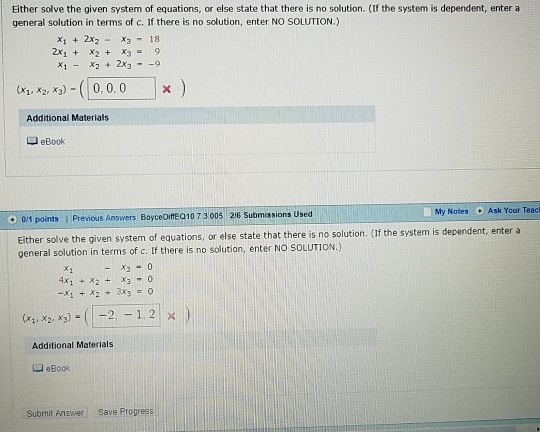3 4 Please help me solve the following Differential Equations problem Either solve the given system of equations, or else state that there is no solution. (If the system is dependent, enter a general solution in terms of c. If there is no solution, enter NO SOLUTION.) x2x2 X3 18 2x1 X2 X39 X1x22x39 (xx0,0.0x Additional Materials a eBook Ask Your Teacl Mty Notes O 0/1 points Previous Answers BoyceDifE 010 7 3005 26 Submissions Used If the system is...

• ### This is Differential equations, please help me, solve and show step by step. MAT 204 Elementary...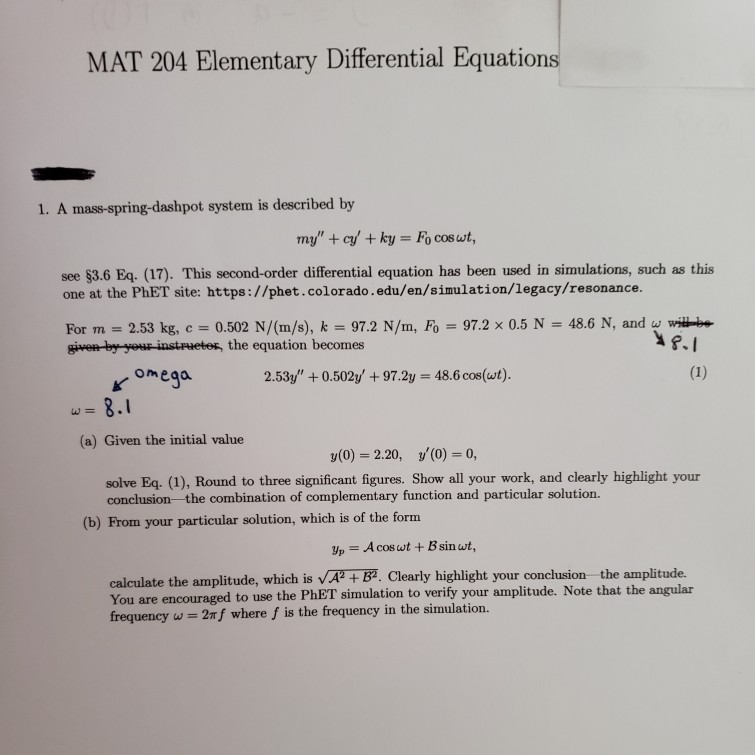This is Differential equations, please help me, solve and show step by step. MAT 204 Elementary Differential Equations 1. A mass-spring-dashpot system is described by my" + cy' + ky = Fo coswt, see \$3.6 Eq. (17). This second-order differential equation has been used in simulations, such as this one at the PhET site: https://phet.colorado.edu/en/simulation/legacy/resonance. = 48.6 N, and w will be For m = 2.53 kg, c = 0.502 N/(m/s), k 97.2 N/m, Fo = 97.2 x 0.5 N...

• ### Section 3.2 The Wronskian: Problem 5 Previous Problem Problem List Next Problem (1 point) Determi...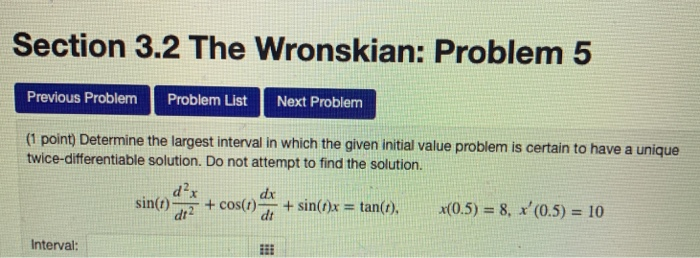Section 3.2 The Wronskian: Problem 5 Previous Problem Problem List Next Problem (1 point) Determine the largest interval in which the given initial value problem is certain to have a unique twice-differentiable solution. Do not attempt to find the solution. d2x sin(t)atx + cos(r)ar + sin(,)x = tan(t), dx x(0.5)-8, x,(0.5)-10 Interval Section 3.2 The Wronskian: Problem 5 Previous Problem Problem List Next Problem (1 point) Determine the largest interval in which the given initial value problem is certain to...

• ### 2. please help me with the following linear algebra question. must show work. Graph the system...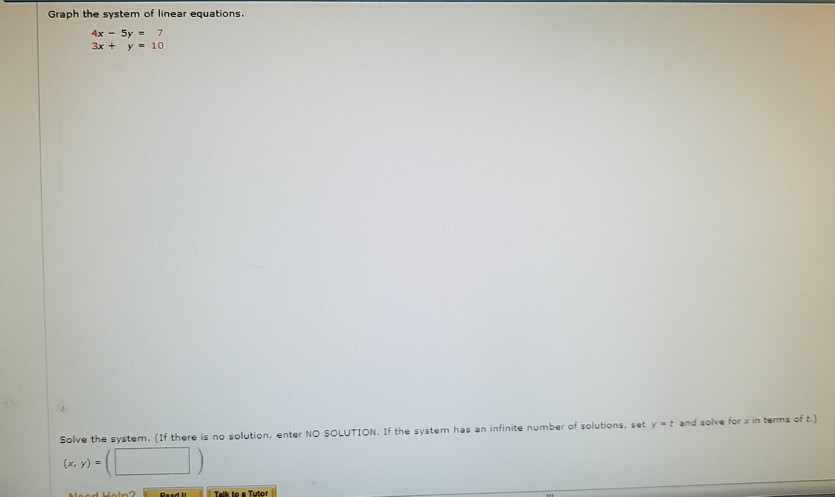2. please help me with the following linear algebra question. must show work. Graph the system of linear equations 4x-5y = Solve the system. (If there is no solution, enter NO SOLUTION. If the x, y) - system has an infinite number of solutions, set y- and solve for x in terma of t:)

• ### Hello! I need help with this college level differential equations question. Please show work and ...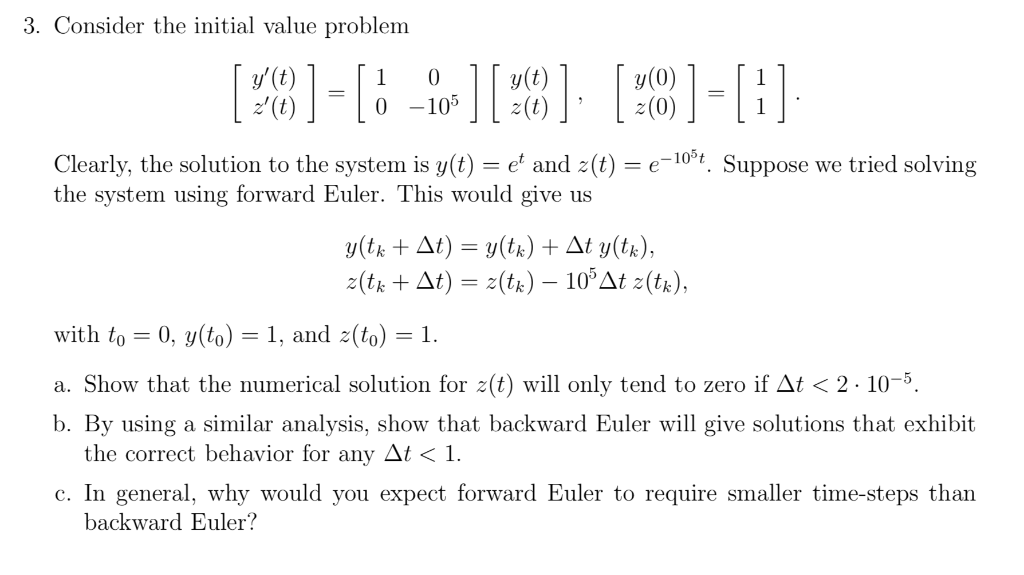Hello! I need help with this college level differential equations question. Please show work and thank you. 3. Consider the initial value problem y' (t) 1 0y(t) y(0) Clearly, the solution to the system is y(t) = et and 2(t) = e-10 t. Suppose we tried solving the system using forward Euler. This would give us with to 0, y(to) 1, and z(to-1. a. Show that the numerical solution for z(t) will only tend to zero if Δι < 2...